# Identify Shapes Using Machine Learning on Arduino Hardware

This example shows how to use the Simulink® Support Package for Arduino® Hardware to identify shapes such as a triangle and circle using a machine learning algorithm. The model in this example is deployed on an Arduino Nano 33 IoT hardware board with an onboard LSM6DS3 IMU Sensor.

You can hold the Arduino board in the palm of your hand and draw the shape in the air. The inertial measurement unit (IMU) sensor captures the linear acceleration and angular rate data along the X-, Y-, and Z- axes. You send this data to the machine learning algorithm, which identifies the shape you have drawn and transmits the output to the serial port of the Arduino hardware. The shape identified by the machine learning algorithm then displays in the MATLAB® Command Window.

### Required Hardware

• Use an Arduino board with an onboard IMU sensor. This example uses Arduino Nano 33 IoT that has an onboard LSM6DS3 IMU sensor. This helps you to easily hold the hardware in your hand while you draw shapes in the air. Alternatively, you can connect an IMU sensor to any Arduino board that has a sufficiently large memory. For more information on how to connect an IMU sensor to your Arduino board, refer to the sensor datasheet.

• USB cable

### Hardware Setup

Connect the Arduino Nano 33 IoT board to the host computer using the USB cable.

### Capture Data Set for Training Machine Learning Algorithm

This example provides you with the MAT file `shapes_training_data.mat` containing the data set for the circle and triangle shapes.

Run this command in the MATLAB Command Window to load this file in MATLAB.

```load shapes_training_data ```

The `shapes_training_data` MAT file contains 119 data samples that are read from the accelerometer and gyroscope on the IMU sensor. These 119 samples are grouped into 100 frames, with each frame representing a hand gesture. Each frame has six values that are obtained from the X-, Y-, and Z- axes of the accelerometer and gyroscope, respectively. A total of 11,900 observations are stored in the data set for the circle and triangle shapes.

The `cature_training_data.m` file captures the training data for the `arduino_machinelearning` model. You can train the model using preconfigured MATLAB code in the `capture_training_data.m` file and store the trained data in the `shapes_training_data.mat` file.

This example uses the `gr_script_shapes.m` file to preprocess the data set for the circle and triangle shapes, train the machine learning algorithm with the data set, and evaluate algorithm's ability to accurately predict the shapes. Follow this procedure to train and store the data in the MAT file `shapes_training_data.mat`.

1. In the MATLAB Command Window, run this command to edit the `gr_script_shapes.m` file. The MATLAB function in this file loads data from the `shapes_training_data.mat` file, preprocesses and trains the data, and then performs a five-fold cross-validation for an ensemble classifier and compute its validation accuracy.

```edit gr_script_shapes; ```

2. Configure these parameters.

a. Se the acceleration threshold for movement detection. Specify this value in the `accelerationThreshold` parameter. In this example, the threshold is set to `2.5`. For more information on how to adjust the acceleration threshold for an IMU sensor, refer to the sensor datasheet.

b. To read data from the LSM6DS3 IMU sensor, create an `lsm6ds3 (MATLAB Support Package for Arduino Hardware)` object and specify the number of samples read in a single execution of the read function. In this example, the parameter is set to `119`.

c. Specify the number of frames to be captured per gesture in the `while` loop. In this example, `100` frames are captured per gesture.

3. Hold the Arduino hardware in the palm of your hand and draw a circle in the air. If you want to create a data set of 100 frames for a circle, draw a circle 100 times in the air. Run this command in the MATLAB Command Window.

```circle_data = shapes_training_data; ```

In the MATLAB Command Window, observe the value of the Gesture no. variable rising each time you draw a circle.

4. Similarly, for a triangle, hold the Arduino hardware in the palm of your hand and draw a triangle in the air. Run this command in the MATLAB Command Window.

```triangle_data = shapes_training_data; ```

5. When you create your own data set for the circle and triangle shapes, use the same name for the MAT file in the `gr_script_shapes` MATLAB code file. The `gr_script_shapes` MATLAB code file is used to preprocess and train the data set for a circle and a triangle, train the machine learning algorithm with the data set, and evaluate its performance to accurately predict these shapes.

### Extract Feature

The machine learning algorithm used in this example requires features that are extracted by taking the mean and the standard deviation of each column in a frame. Considering the X-, Y-, and Z- axes data for accelerometer and gyroscope each, this results in a 100-by-12 matrix of observations for each gesture.

`Nframe = 100;`
```for ind = 1:Nframe featureC1(ind,:) = mean(circledata{ind}); featureC2(ind,:) = std(circledata{ind});```
``` featureT1(ind,:) = mean(triangledata{ind}); featureT2(ind,:) = std(triangledata{ind}); end```
```X = [featureC1,featureC2; featureT1,featureT2; zeros(size(featureT1)),zeros(size(featureT2))];```
```% labels - 1: circle, 2: triangle, 3: idle Y = [ones(Nframe,1);2*ones(Nframe,1);3*ones(Nframe,1)];```

### Prepare Data

This example uses 80% of the observations to train a model that classifies two types of shapes and 20% of the observations to validate the trained model. Use `cvpartition` (Statistics and Machine Learning Toolbox) to hold out 20% of the data for the test data set.

```rng('default') % For reproducibility Partition = cvpartition(Y,'Holdout',0.20); trainingInds = training(Partition); % Indices for the training set XTrain = X(trainingInds,:); YTrain = Y(trainingInds); testInds = test(Partition); % Indices for the test set```

In MATLAB, the Workspace pane is populated with these parameters.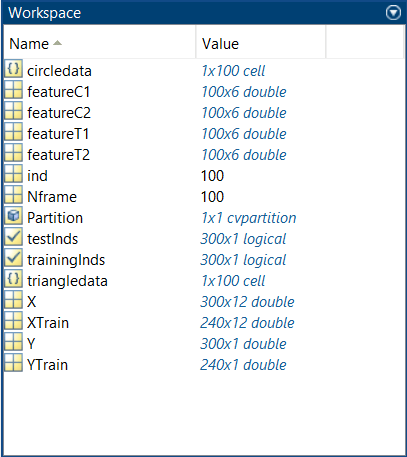### Train Classification Model Using Classification Learner App

The Classification Learner app helps you explore supervised machine learning using various classifiers. Using this app, you can explore your data, select features, specify validation schemes, train models, and assess results. You can perform automated training to find the best classification model for your application. You can perform supervised machine learning by supplying a known set of input data (observations or examples) and known responses to the data (such as labels or classes). You use this data to train a model that generates predictions for the response to new data. To use the model with new data, or to learn about programmatic classification, you can export the model to the workspace or generate MATLAB code to re-create the trained model. For more information, see the Classification Learner App.

1. To open the Classification Learner app, enter `classificationLearner` in the MATLAB Command Window. You can also find the app on the Apps tab, under Machine Learning.

2. On the Classification Learner tab, in the File section, click New Session > From Workspace.

3. In the New Session from Workspace dialog box, under Data Set Variable, select a `XTrain`.

4. Under Response, select From Workspace and select `YTRain`.

5. In the Validation section, select Cross-Validation. The default validation option is five-fold cross-validation, which protects against overfitting. For more information on how to select and validate data for a classification problem, see Select Data and Validation for Classification Problem.

For more information on choosing the best classification model and avoiding overrifting, see Machine Learning Challenges.

6. In the Test section, select Set aside test data set and set Test Data Percent to `20`.

7. Click Start Session.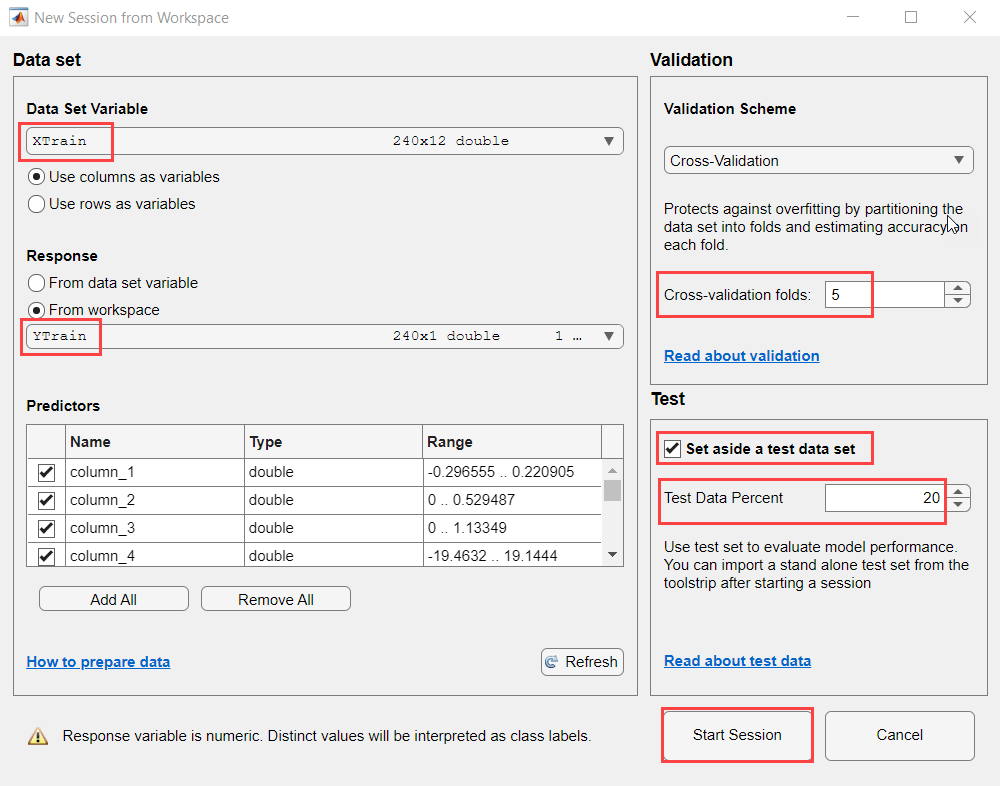8. Choose features to plot using the X and Y lists under Predictors. You can add or remove predictors using the check boxes in the Classes section.

### Train Classification Model Using Ensemble Classifier

This section describes how to train the classification model using an ensemble classifier. You can use any classifier that provides results with the highest accuracy for the trained data. For more information on how to train models, select features, and evaluate results, see Train Classification Models in Classification Learner App.

1. In the top pane of the Classification Learner app, in the Models section, select `Optimizable Ensemble`.

Note: This model performs hyperparameter optimization during training, which can result in a model with higher accuracy than one of the other ensemble classifiers. For more information, see Hyperparameter Optimization in Classification Learner App (Statistics and Machine Learning Toolbox).

2. In the Train section, clear Use Parallel and then select Train All > Train Selected. Observe the Accuracy (Validation) of the Ensemble classifier and the Minimum Classification Error Plot tab.

Note: Clear the Use Parallel option so that you can view the Minimum Classification Error Plot while the hyperparameter optimization is in progress. You can run the optimization in parallel, but you cannot view the Minimum Classification Error Plot until the hyperparameter optimization is complete.Note: Since hyperparameter optimization uses a random optimization method and the optimization problem is highly nonlinear, you can end up with different set of hyperparameters and slightly different accuracy.

3. In the Test section, select Test All > Test Selected. Observe that the accuracy of the ensemble classifier for testing the data is 100%.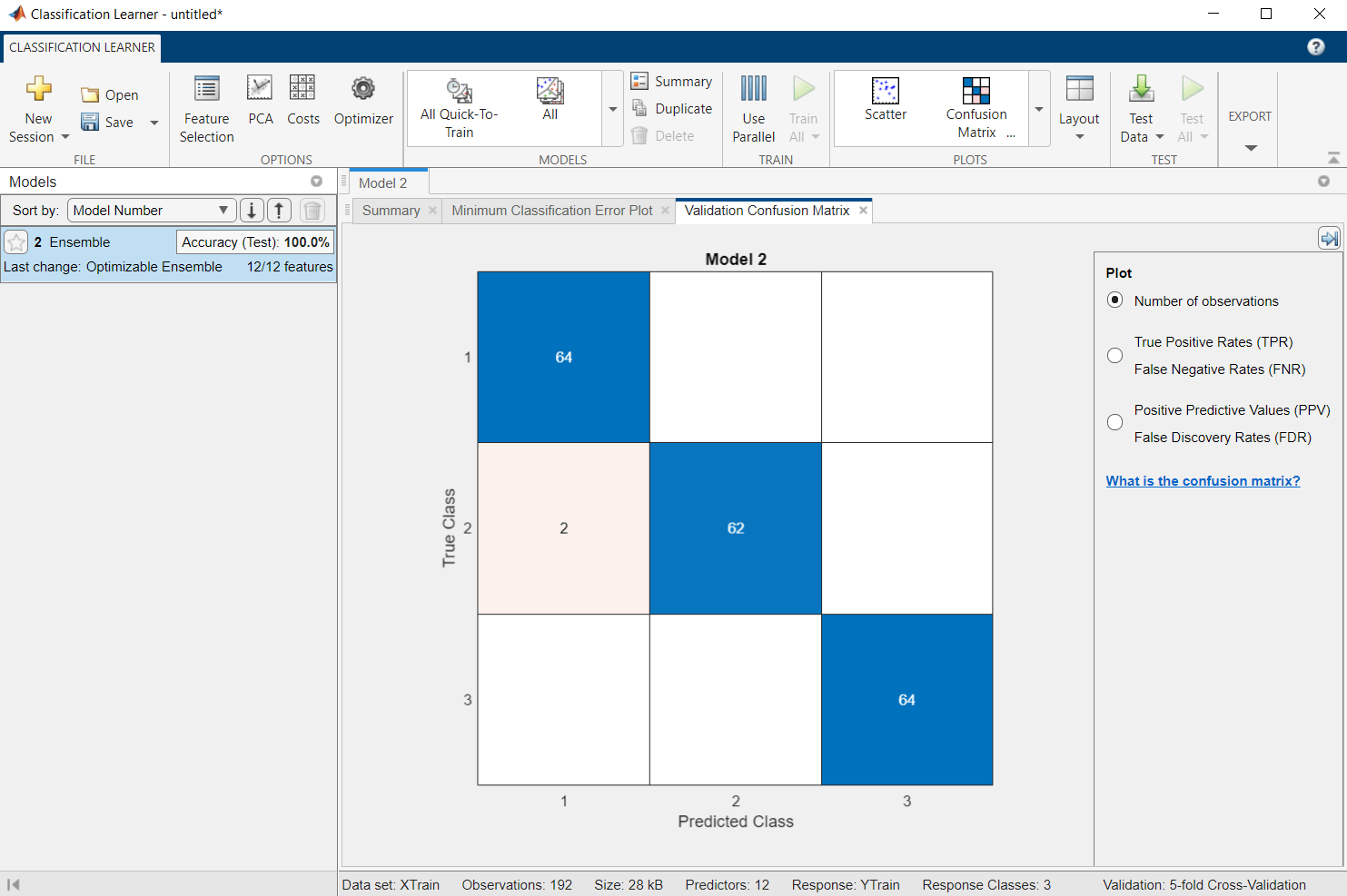4. Click Export > Export Model. In the Export Model dialog box, enter the workspace variable name of the trained model. In this example, it is set to `ensMdl`. Click OK.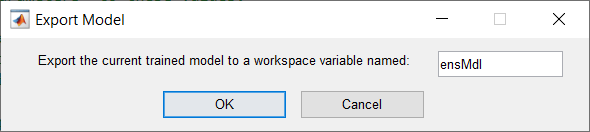For more information, see Export Classification Model to Predict New Data.

In MATLAB, under Workspace, observe that the `ensMdl` parameter is now visible.The trained model accurately classifies 100% of the shapes in the test data set. This result confirms that the trained model does not overfit the training data set.

### Prepare Simulink Model and Calibrate Parameters

Use `gr_script_shapes.m` file as a Simulink model initialization function.

To open the Simulink model, run this command in the MATLAB Command Window.

```open_system('arduino_machinelearning_shapes') ```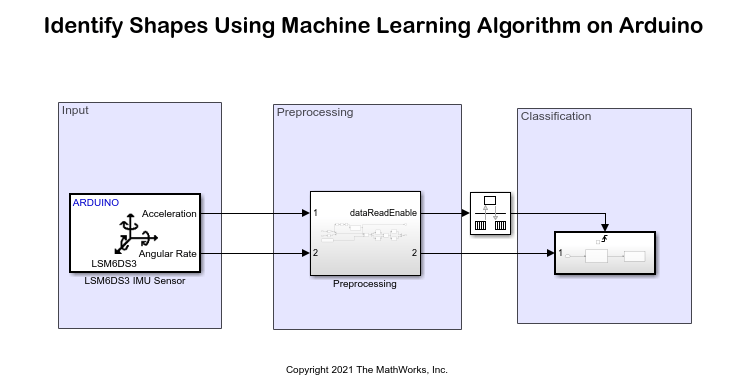The Arduino Nano 33 IoT board has an onboard LSM6DS3 IMU sensor that measures linear acceleration and angular velocity along the X-, Y-, and Z-axes. Configure these parameters in the Block Parameters dialog box of the LSM6DS3 IMU Sensor block.

1. Set the I2C address of the sensor to `0x6A` to communicate with the accelerometer and gyroscope peripherals of the sensor.

2. Select the Acceleration (m/s^2) and Angular velocity (rad/s) output ports.

3. Set the Sample time to `0.01`.

The 1-by-3 acceleration and angular velocity vector data is collected from the LSM6DS3 IMU sensor at the sample time you specify in the Block Parameters dialog box. The Preprocessing subsystem then preprocesses this data.

To open the subsystem, run this command in the MATLAB Command Window.

```open_system('arduino_machinelearning_shapes/Preprocessing') ```The acceleration data is first converted from m/s^2 to g. The absolute values are then summed and for every 119 data values that are greater than a threshold of 2.5 g, the dataReadEnable parameter in the MATLAB Function block becomes logically true. A true value acts as a trigger to the Triggered subsystem in the Classification area. This threshold of 2.5 g is the value you set as the acceleration threshold parameter in the `gr_script_shapes.m` file.

The angular velocity data is converted from radians to degrees. The acceleration and angular velocity data is multiplexed and given as an input to the switch. For a data value greater than 0, the buffer stores the valid 119 gesture values corresponding to a circle and a triangle. For data values less than zero, which indicates that no hand gesture is detected, a series of [1,6] zeros are sent to the output to match the combined acceleration and angular velocity data.

Set the Output buffer size parameter to `119` in the Buffer block.

Features are extracted by calculating the mean and the standard deviation values of each column in a frame that results in a 100-by-12 matrix of observations for each gesture. These extracted features are further passed as an input to the Triggered subsystem in the Classification area.

To open the subsystem, run this command in the MATLAB Command Window.

```open_system('arduino_machinelearning_shapes/Triggered Subsystem') ```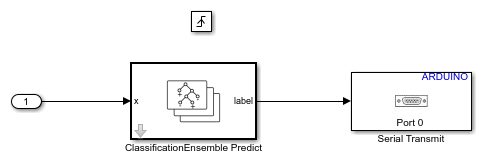The Rate Transition block transfers data from the output of the Preprocesssing subsystem operating at one rate to the input of the Triggered subsystem operating at a different rate.

In the ClassificationEnsemble Predict (Statistics and Machine Learning Toolbox) block, set Select trained machine learning model to the variable name that you set while exporting the trained classification model from the Classification Learner app. Enter the same workspace variable name of the trained model as in the Classification Learner app. In this example, it is set to `ensMdl`.

The Serial Transmit block parameters are configured to their default values.

### Deploy Simulink Model on Arduino

1. On the Hardware tab of the Simulink model, in the Mode section, select Run on board and then click Build, Deploy & Start.

Note: For more information on how to troubleshoot a deployment error for a larger memory footprint of the code deployed on your Arduino board, see Troubleshoot Deployment Error for Code with Large Memory Footprint section in this example.

2. For easy analysis of the hand movement data recognized by the machine learning algorithm, run the following script in the MATLAB Command Window and read data on the Arduino serial port.

```device = serialport(serial_port,9600); while(true) %Read double value rxData = read(device,1,"double"); if rxData == 1 disp('Circle'); elseif rxData == 2 disp('Triangle'); end end```

You can also run this command in the MATLAB Command Window and edit the `read_shapes_data_from_device.m` file.

`edit read_shapes_data_from_device;`

3. Replace the serialport parameter with the actual com port of your Arduino board. The gesture detected by the machine learning algorithm is displayed on the Arduino serial port at a baud rate of 9600.

4. Hold the hardware in the palm of your hand and draw shapes in the air. Observe the output in the MATLAB Command Window.### Troubleshoot Deployment Error for Code with Large Memory Footprint

Based on the classification model you select, the code deployed on your Arduino board varies in size. If this code is larger than the memory of your Arduino board, this error message is displayed in the Diagnostic Viewer window.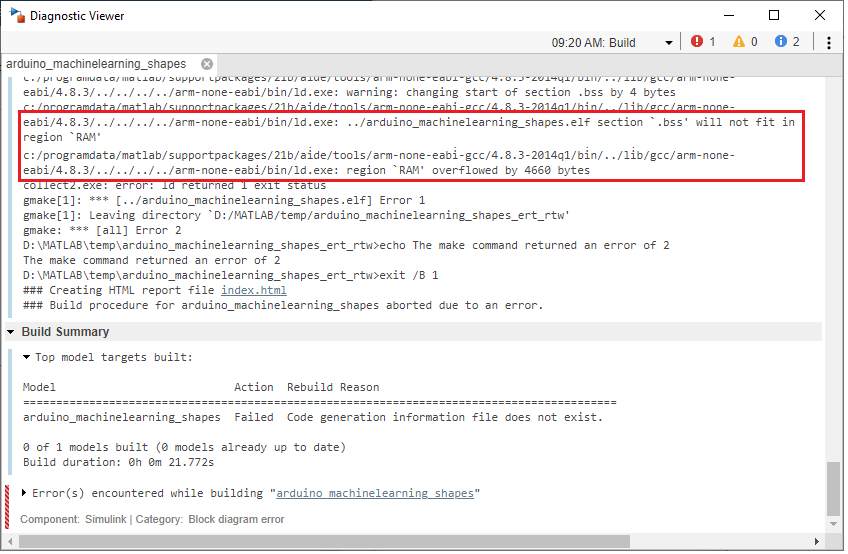You can troubleshoot this error by implementing one of the following methods:

• Change the data type of the classifier block to single precision and observe the impact on the model size and accuracy of output.If you do switch to a different classifier model, update the precision of the classifier in the block mask accordingly.

### Other Things to Try

• Train the machine learning algorithm to identify shapes such as squares, pentagons, and numbers from 0 to 9.

• Try models other than ensemble classifier used in this example, especially if you switch to a different classification task. The machine learning block library in Simulink covers SVMs, decision trees, and Gaussian processes, aside from the ensemble and neural network classifiers. For more information on different classifiers, see Statistical and Machine Learning Toolbox blocks.